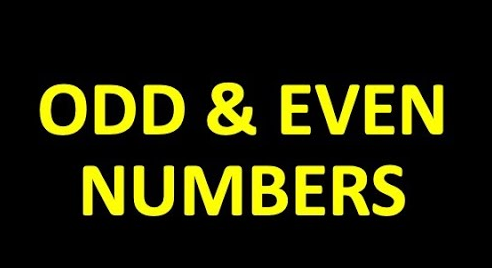# Describe if statement. Write an if statement to multiply even numbers by 3 and odd numbers by 4.The if statement is a conditional statement in programming that allows a program to make decisions based on certain conditions. It takes a condition and executes a block of code if the condition is true. If the condition is false, the code block is skipped.

To multiply even numbers by 3 and odd numbers by 4 using an if statement, we can use the modulo operator (%) to check if a number is even or odd. The modulo operator returns the remainder of a division operation.

Here’s an example if statement in C programming language:

int number = 10;

if (number % 2 == 0) {

number = number * 3; // multiply even numbers by 3

} else {

number = number * 4; // multiply odd numbers by 4

}

In this example, the if statement checks if the number is even by checking if the remainder of the number divided by 2 is equal to 0. If it is, the number is multiplied by 3. If not, the number is multiplied by 4.

This can be applied to any natural number by using an appropriate variable to store the value of the number.